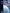# Is a textbook a secondary source?

It depends. A textbook can either be a secondary or tertiary source and, in seldom cases, a primary source. In most cases, the author of a textbook interprets prescribed theories of a topic and would, therefore, be a secondary source. A textbook can be a tertiary source when it simply indexes information about a particular topic. Finally, if you were to research the development of textbooks during a specific time period, then a textbook could be used as a primary source.

For example, the IB Mathematical Studies textbook is to be used as a secondary source in any research as its authors are not the original authors of the theories, but they use their own words and perspective to describe them.IB Math Studies as an example of a textbook as secondary source.

If this textbook resembled a dictionary, then it would have to be referenced as a tertiary source. To give an idea, if it did not have any textual reference, like an introduction or explanations of mathematical theories, then it would be a tertiary source. Still, it could be referenced as a primary source in a study about textbooks for IB diploma students in the last 10 years.

🧪 Is a science textbook a secondary source?

It depends. A science textbook can either be a secondary or tertiary source and, in seldom cases, a primary source. In most cases, the author of a textbook interprets prescribed theories of a topic and would, therefore, be a secondary source.

📗 When is a textbook a tertiary source?

A textbook can be a tertiary source when it simply indexes information about a particular topic.

🥇 When is a textbook a primary source?

if you were to research the development of textbooks during a specific time period, then a textbook could be used as a primary source.

🧲 Is a physics textbook a secondary source?

It depends. A physics textbook can either be a secondary or tertiary source and, in seldom cases, a primary source. In most cases, the author of a textbook interprets prescribed theories of a topic and would, therefore, be a secondary source.

🤯 Is a psychology textbook a secondary source?

It depends. A psychology textbook can either be a secondary or tertiary source and, in seldom cases, a primary source. In most cases, the author of a textbook interprets prescribed theories of a topic and would, therefore, be a secondary source.